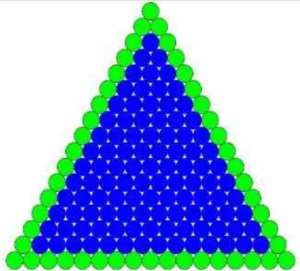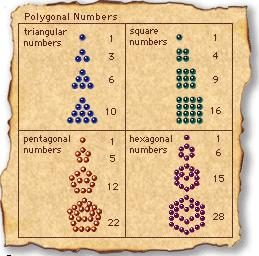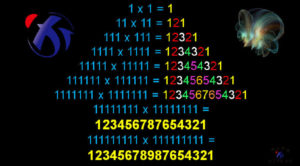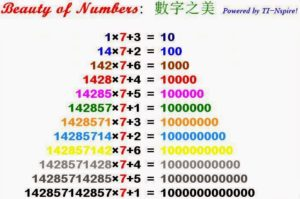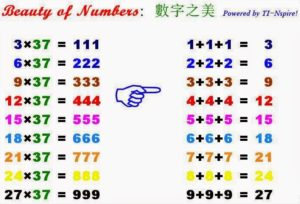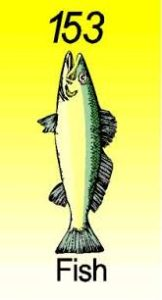# 聖經裏真有密碼么？從約翰福音的密碼153看: 一、聖父上帝編寫密碼, 二、聖子上帝啟示密碼, 三、聖靈上帝解釋密碼 (何治平牧師證道)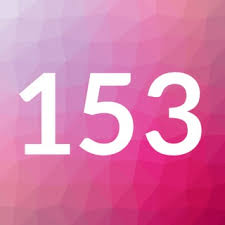A、創世記第一章第二章

B、142857X7＝999999

A、基數的數根

B、根據三一函數歸根法，

A、數字的屬靈意義 2/3=0.666

B、天上和地上的三分之一

1、2、3、4、5、6、7 各除以 7
1/7 = 0.142857142857……
2/7 = 0.285714285714……
3/7 = 0.428571428571……
4/7 = 0.571428571428……
5/7 = 0.714285714285……
6/7 = 0.857142857142……

1、2、3、4、5、6日预表世界历史6千年

7/7 = 1 唯一整数定为圣日

3：  3x3x3= 27
2x2x2 + 7x7x7 = 351
3x3x3 + 5x5x5 + 1x1x1= 153
1x1x1 + 5x5x5 + 3x3x3= 153

6：  6x6x6=216
2x2x2 + 1x1x1 + 6x6x6= 225
2x2x2 + 2x2x2 + 5x5x5= 141
1x1x1 + 4x4x4 + 1x1x1= 66
6x6x6 + 6x6x6 = 432
4x4x4 + 3x3x3 +2x2x2=99
9x9x9 + 9x9x9 = 1458
1x1x1 + 4x4x4 + 5x5x5+8x8x8 = 702
7x7x7 + 2x2x2 = 351
3x3x3 + 5x5x5 + 1x1x1=153
1x1x1 + 5x5x5 + 3x3x3=153

9：9x9x9=729
7x7x7+2x2x2+9x9x9=1080
1x1x1+8x8x8=513
5x5x5+1x1x1+3x3x3=153
1x1x1+5x5x5+3x3x3=153

1 的数根 是 1
2 ——-   是 371
3 ——-   是 153
4 ——-   是 133、55、250
5 ——-   是 371
6 ——-   是 153
7 ——-   是 370
8 ——-   是 371
9 ——-   是 153
10——– 是 1
12——– 是153
12000— 是153
144000–是153

> 142857 x 1 = 142857
> 142857 x 2 = 285714
> 142857 x 3 = 428571
> 142857 x 4 = 571428
> 142857 x 5 = 714285
> 142857 x 6 = 857142
> 也是同样的数字，调换了位置，反复的出现。
> 数字 1 4 2 8 5 7  中也没有 3、6、9
> 142857 x 7 = 999999 数根是 153
> 7/7 = 1，代表 1000年
> 第7日 第7千年，万物复兴的千禧年国度
> 代表众圣徒都在千禧年国度里与主一同作王
> 第七位天使吹响第七支号筒时
> 天上就有大聲音說
> 世上的国成了我主和主基督的国
> 祂要作王直到永永遠遠。

2/3=0.666……